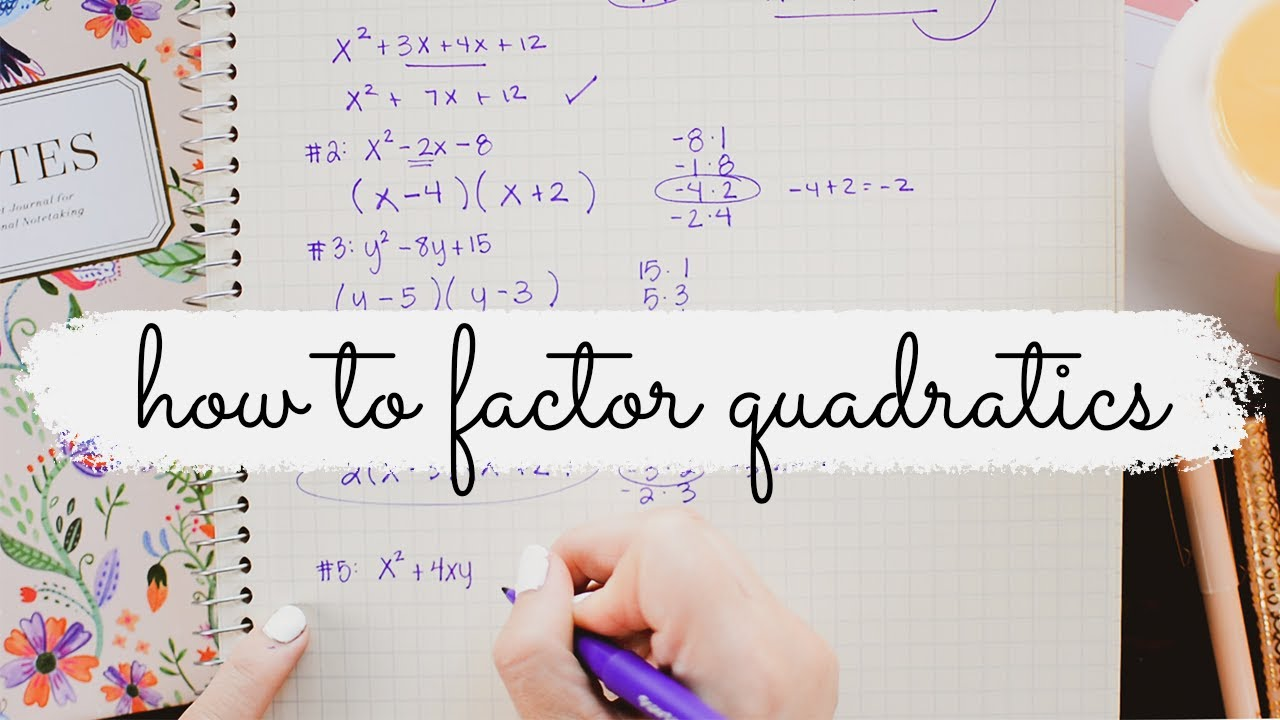#Does one really need to learn how to factorize quadratic? Well, if you plan on studying math even at the school level, then yes, it is really important to learn about it! All of this comes under algebra, and getting proficient in algebra is very important to properly understand maths. With websites such as International Home Study, studying maths can be more flexible then ever.

When we talk about senior mathematics (higher than the normal level), then one of the most important skills which are often used is factoring. Using it, one can understand mathematical concepts and solve difficult problems. In simple words, factoring makes it easy for us to understand difficult equations.

Using factoring, we can rewrite polynomials in a much simpler form, and when we use the factoring principles in equations, this provides us with a lot of information.

When we talk about quadratic equations, their use-case can be found in our everyday life, whether you notice it or not! Do you want to find the speed of a moving object? Do you want to find out the profit of a product? All of these can be achieved by the quadratic formula, and when we combine the skill of factorization, a lot of math and real-life problems can be easily solved!

Do I Really Need to Learn How to Factorise Quadratics?

As mentioned earlier, it is important to know about factoring and quadratic equations as it is an important part of Algebra, and Allegra is an important part of math! But does the usefulness of all this knowledge is only confined inside the classroom and your coursework? That’s not true at all…

So let’s look at the benefits of why you should learn how to factorize quadratics:

Algebraic Manipulation

You can easily manipulate algebraic expressions by learning how to factorize quadratics! If we remove the knowledge of how to factor quadratics, all that’s left in algebra is how to solve simple equations (linear) with 1-2 variables at best. So if you want to learn how to solve advanced algebraic expressions, then you got to learn this stuff.

Complicated Equations

Another benefit is that you can convert complicated equations into simple ones! And since the quadratic formula and factorization are so simple (once learned), you can solve a lot of problems using it! Furthermore, you can also save valuable time by turning rather difficult and complicated equations into simple ones!

As mentioned earlier, the mathematic problems get more difficult as you advance in this field. This means that if you plan a career in mathematics, then it would not be possible to do so without learning algebra. And algebra can’t be learning about factoring quadratic. In fact, it is also a part of the IGCSE!

Find Area of Room

Did you know that you can also find the area of a room or any piece of land using the quadratic equations? As you can see, there are tons of real-life applications of this mathematical concept which can prove to be really useful even later in life.

Speed Calculation

Quadratic equations can also be used for speed calculations. For example, it is common among the kayakers to find their speed when going down/up a river using the quadratic equations!